# Plotting Distributions with Seaborn#

With Seaborn, it is also very practical to plot data distributions such as boxplots, bar graphs, histograms and kernel density estimation plots.

import pandas as pd
import numpy as np
import matplotlib.pyplot as plt
import seaborn as sns


We start by loading a table of measurements into a pandas DataFrame.

df = pd.read_csv("../../data/BBBC007_analysis.csv")
df

area intensity_mean major_axis_length minor_axis_length aspect_ratio file_name
0 139 96.546763 17.504104 10.292770 1.700621 20P1_POS0010_D_1UL
1 360 86.613889 35.746808 14.983124 2.385805 20P1_POS0010_D_1UL
2 43 91.488372 12.967884 4.351573 2.980045 20P1_POS0010_D_1UL
3 140 73.742857 18.940508 10.314404 1.836316 20P1_POS0010_D_1UL
4 144 89.375000 13.639308 13.458532 1.013432 20P1_POS0010_D_1UL
... ... ... ... ... ... ...
106 305 88.252459 20.226532 19.244210 1.051045 20P1_POS0007_D_1UL
107 593 89.905565 36.508370 21.365394 1.708762 20P1_POS0007_D_1UL
108 289 106.851211 20.427809 18.221452 1.121086 20P1_POS0007_D_1UL
109 277 100.664260 20.307965 17.432920 1.164920 20P1_POS0007_D_1UL
110 46 70.869565 11.648895 5.298003 2.198733 20P1_POS0007_D_1UL

111 rows × 6 columns

## Boxplots#

The axes function for plotting boxplots is boxplot.

Seaborn already identified file_name as a categorical value and ìntensity_mean as a numerical value. Thus, it plots boxplots for the intensity variable. If we invert x and y, we still get the same graph, but as vertical bosplots.

sns.boxplot(data=df, x="intensity_mean", y="file_name")

<AxesSubplot: xlabel='intensity_mean', ylabel='file_name'>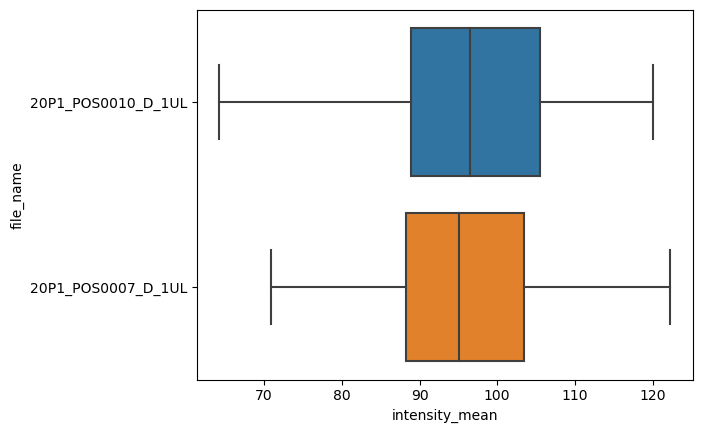The figure-level, and more general, version of this kind of plot is catplot. We just have to provide kind as box.

sns.catplot(data=df, x="intensity_mean", y="file_name", kind="box")

<seaborn.axisgrid.FacetGrid at 0x27775d754f0>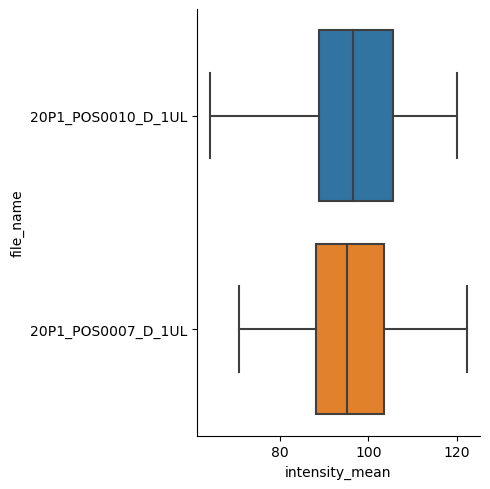There are other kinds available, like a bar graph.

sns.catplot(data=df, x="file_name", y="intensity_mean", kind="bar")

<seaborn.axisgrid.FacetGrid at 0x2777b1abb80>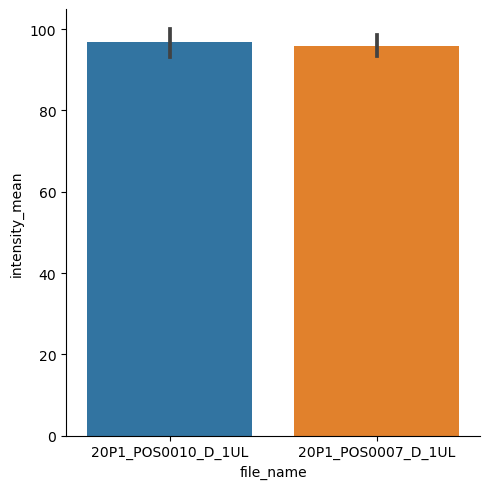## Histograms and Distribution Plots#

The axes-level function for plotting histograms is histplot.

sns.histplot(data = df, x="intensity_mean", hue="file_name")

<AxesSubplot: xlabel='intensity_mean', ylabel='Count'>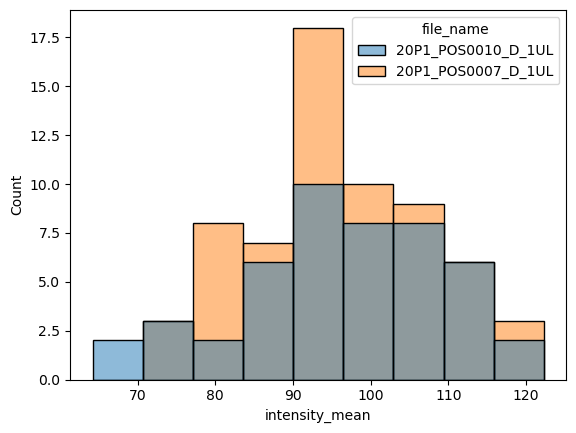We can instead plot the kernel density estimation (kde) with kdeplot function. Just be careful while interpreting these plots (check some pitfalls here)

sns.kdeplot(data=df, x="intensity_mean", hue="file_name")

<AxesSubplot: xlabel='intensity_mean', ylabel='Density'>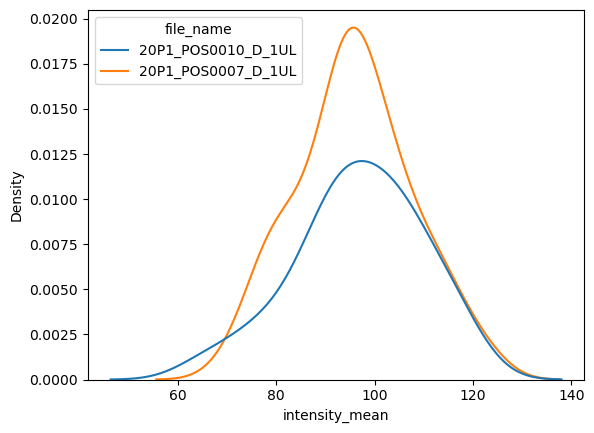The figure-level function for distributions is distplot. With it, you can have histograms and kde in the same plot, or other kinds of plots, like the empirical cumulative distribution function (ecdf).

sns.displot(data = df, x="intensity_mean", hue="file_name", kde=True)

<seaborn.axisgrid.FacetGrid at 0x2777b77c910>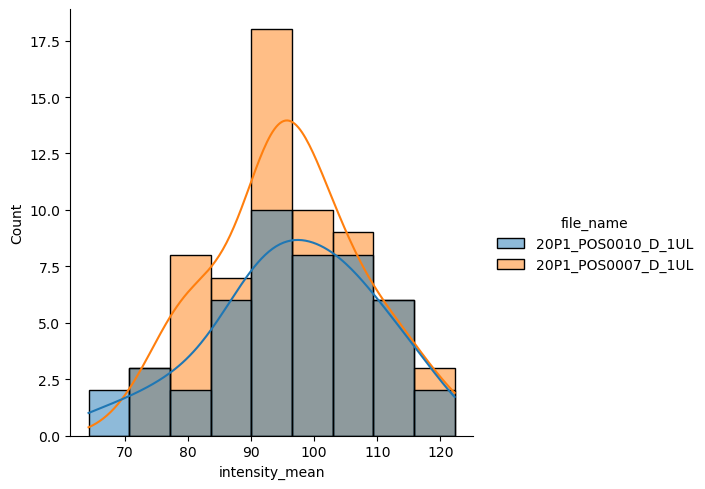## Exercise#

Plot two empirical cumulative distribution functions for ‘area’ from different files on a same graph with different colors.

Repeat this for the property ‘intensity_mean’ on a second figure. Infer whether you would expect these properties to be different or not.

*Hint: look for the kind parameter of displot